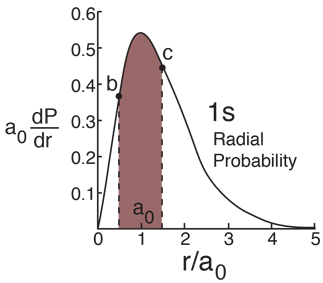# Probability for a Range of RadiusHydrogen Ground StateFinding the probability that the electron in the hydrogen ground state will be found in the range r=b to r=c requires the integration of the radial probability density.This requires integration by parts. The form of the solution isFor a range from b = a0 to c= a0
the probability is x 10^.